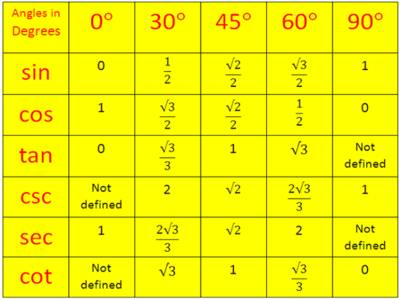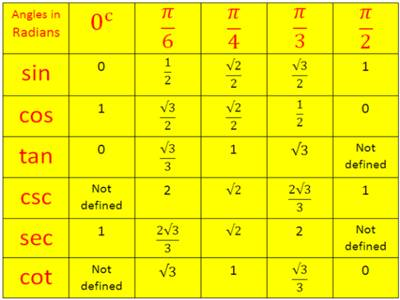“Trigon” is Greek for the triangle, and “metric” is Greek for measurement. The trigonometric ratios are special measurements of a right triangle (a triangle with one angle measuring 90°). Remember that the two sides of a right triangle which form the right angle are called the legs, and the third side (opposite the right angle) is called the hypotenuse.

Trigonometrical Ratios Table

The Trigonometrical ratios table will help us to find the values of trigonometric standard angles.

The standard angles of trigonometrical ratios are 0°, 30°, 45°, 60° and 90°.

The values of trigonometrical ratios of standard angles are very important to solve the trigonometrical problems. Therefore, it is necessary to remember the value of the trigonometrical ratios of these standard angles. The sine, cosine, and tangent of the standard angles are given below in the table.

Trigonometric Table in Sexagesimal SystemTrigonometric Table in Circular SystemNote: Values of sin θ and cos θ lies between 0 and 1 (both inclusive)

To remember the above values:

(a) divide the numbers 0, 1, 2, 3 and 4 by 4,

(b) take the positive square roots,

(c) these numbers given the values of sin 0°, sin 30°, sin 45°, sin 60° and sin 90° respectively.

(d) write the values of sin 0°, sin 30°, sin 45°, sin 60° and sin 90° in reverse order and get the values of cos 0°, cos 30°, cos 45°, cos 60° and cos 90° respectively.

If θ be an acute angle, the values of sin θ and cos θ lies between 0 and 1 (both inclusive).

The sine of the standard angles 0°, 30°, 45°, 60° and 90° are respectively the positive square roots of 0/4,1/4, 2/4,3/4 and 4/4

Therefore,

sin 0° = √(0/4) = 0

sin 30° = √(1/4) = ½

sin 45° = √(2/4) = 1/√2 = √2/2

sin 60° = √3/4 = √3/2;

cos 90° = √(4/4) = 1.

Similarly  cosine of the above standard angels are respectively the positive square roots of 4/4, 3/4, 2/4, 1/4, 0/4

Therefore,

cos 0° = √(4/4) = 1

cos 30° = √(3/4) = √3/2

cos 45° = 1

cos 60° = √(1/4) = 1/2

cos 90° = √(0/4) = 0.

Since, we know the sin and cos value of the standard angles from the trigonometrical ratios table; therefore we can easily find the values of the other trigonometrical ratios of the standard angles.

The tangent of the standard angles 0°, 30°, 45°, 60° and 90°:

tan 0° = 0

tan 30° = √3/3

tan 45° = √(2/4) = 1/√2 = √2/2

tan 60° = √3

tan 90° = not defined.

The cosine of the standard angles 0°, 30°, 45°, 60° and 90°:

csc 0° = not defined.

csc 30° = 2

csc 45° = √2

csc 60° = 2√3/3

csc 90° = 1.

The secant of the standard angles 0°, 30°, 45°, 60° and 90°:

sec 0° = 1

sec 30° = 2√3/3

sec 45° = √2

sec 60° = 2

sec 90° = not defined.

The cotangent of the standard angles 0°, 30°, 45°, 60° and 90°:

cot 0° = not defined.

cot 30° = √3

cot 45° = 1

cot 60° = √3/3

cot 90° = 0

Information Source: